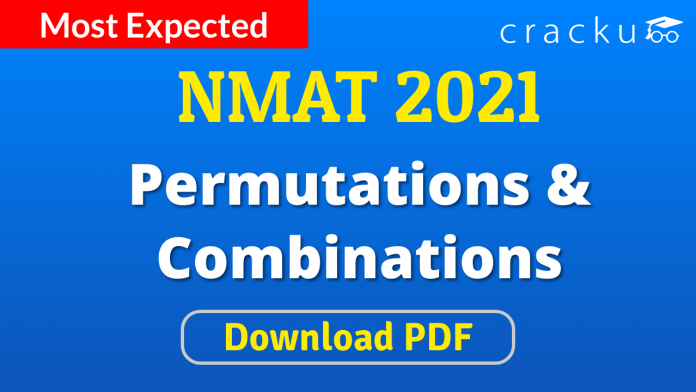# Permutations & Combinations Questions for NMAT

0
1061# Permutations & Combinations Questions for NMAT:

Download Permutations & Combinations Questions for NMAT PDF. Top 10 very important NMAT Permutations & Combinations Questions based on asked questions in previous MBA exam papers.

Take NMAT mock test

Question 1: Letters of the word DIRECTOR are arranged in such a way that all the vowel come together .Find the No of ways making such arrangement?

a) 4320

b) 720

c) 2160

d) 120

e) None of these

Question 2: In a box carrying one dozen of oranges one third have become bad.If 3 oranges taken out from the box random ,what is the probability that at least one orange out of the 3 oranges picked up is good ?

a) 1/55

b) 54/55

c) 45/55

d) 3/55

e) None of these

Question 3: In how many different ways can the letters of the word TRUST’ be arranged?

a) 240

b) 120

c) 80

d) 25

e) None of these

Question 4: How many such pairs of letters are there in the word FOREHAND each of which have as many letters between them in the word as they have in the English alphabet?

a) None

b) One

c) Two

d) Three

e) More than three

Question 5: If it is possible to make only one meaningful word from the second, the fourth, the sixth and the ninth letters of the word PROACTIVE, using each letter only once, second letter of that word is your answer. If more than one word can be formed your answer is M and if no such word can be formed your answer is N.

a) A

b) E

c) T

d) M

e) N

Question 6: In how many different ways can the letters of the word DRASTIC be arranged in such a way that the vowels always come together?

a) 720

b) 360

c) 1440

d) 540

e) None of these

Question 7: Which of the following set of fractions is in ascending order ?

a) 13/15, 11/13, 7/8, 8/9

b) 11/13, 13/15, 7/8, 8/9

c) 8/9, 7/8, 13/15, 11/13

d) 7/8, 8/9, 11/13, 13/15

e) None of these

Question 8: Certain number of pieces of an article are to be packed in boxes, such that each box contains 145 pieces. If after packing them in 32 boxes 25 pieces are left out, what was the number of pieces to be packed ?

a) 4566

b) 4655

c) 4465

d) 4640

e) None of these

Question 9: In how many different ways can the letters of the word ‘HAPPY’ be arranged ?

a) 120

b) 140

c) 60

d) 70

e) None of these

Question 10: In how many different ways can the letters of the word ‘FRANCE’ be arranged ?

a) 2400

b) 720

c) 2005

d) 5040

e) None of these

Word – DIRECTOR

So “I,E,O” are there are 3! ways to arrange the vowels

Now “D,R,C,T,R” are the remaining alphabets ,

Condition is that the vowels should always be together so we can assume the vowels as a single alphabet/unit say “X” (‘X’=’I,E,O’) so now we have a new word – “D,R,C,T,R,X”

Possible arrangements for this word = 6!

Thus total number of ways to rearrange DIRECTOR with vowels grouped together = (Possible arrangements of ‘DRCTRX’) $\times$ (Possible arrangements of vowels)

= 6! $\times$ 3! = $720 \times 6 = 4320$

=> Ans – (A)

Total number of oranges in the box = 12

Number of ways of selecting 3 oranges out of 12 oranges, n(S) = $C^{12}_3$

= $\frac{12 \times 11 \times 10}{1 \times 2 \times 3} = 220$

Number of oranges which became bad = $\frac{12}{3}=4$

Number of ways of selecting 3 oranges out of 4 bad oranges = $C^4_3 = C^4_1 = 4$

Number of desired selection of oranges, n(E) = 220 – 4 = 216

$\therefore$ $P(E) = \frac{n(E)}{n(S)}$

= $\frac{216}{220}= \frac{54}{55}$

=> Ans – (B)

Word = ‘TRUST’

There are 5 letters and ‘T’ is repeated.

If there are ‘n’ letters and ‘r’ are repeating, then number of ways of arranging them = $\frac{n !}{r !}$

$\therefore$ Number of ways in which letters of the word ‘TRUST’ can be arranged

= $\frac{5 !}{2 !} = \frac{5 \times 4 \times 3 \times 2 \times 1}{2 \times 1}$

= $5 \times 4 \times 3 = 60$

=> Ans – (E)

Word = FOREHAND

There are 2 pairs of letters which have as many letters between them in the word as they have in the English alphabet

FA and RN

Word = PROACTIVE

2nd, 4th, 6th and 9th letters = R, A, T, E

No. of words that can be formed by using (R,A,T,E)

= Rate , Tear

Since only 2 words are formed

=> Ans = M

There are 7 letters in the word ‘DRASTIC’ including 2 vowels (A,I) and 5 consonants (D,R,S,T,C).

Considering the two vowels as 1 letter, we have 6 letters which can be arranged in 6! ways

But  corresponding to each way of these arrangements, the vowels can be put together in 2! ways.

Hence, required number of words = $6! \times 2!$

= 720 * 2 = 1440

Decimal equivalent of :

$\frac{13}{15} = 0.87$

$\frac{11}{13} = 0.85$

$\frac{7}{8} = 0.875$

$\frac{8}{9} = 0.89$

Clearly, 0.85 < 0.87 < 0.875 < 0.89

=> $\frac{11}{13} < \frac{13}{15} < \frac{7}{8} < \frac{8}{9}$

No. of pieces

= 32 * 145 + 25

= 4640 + 25 = 4665

The word HAPPY contains 5 letters in which letter ‘P’ comes twice.

=> Number of arrangements = $\frac{5!}{2!}$

= $\frac{120}{2} = 60$